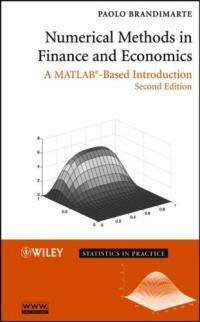> 상세정보

# 상세정보## Numerical methods in finance and economics : a MATLAB-based introduction 2nd ed (41회 대출)

자료유형
단행본
개인저자
Brandimarte, Paolo. Brandimarte, Paolo
서명 / 저자사항
Numerical methods in finance and economics : a MATLAB-based introduction / Paolo Brandimarte.
판사항
2nd ed.
발행사항
Hoboken, N.J. :   Wiley-Interscience ,   c2006.
형태사항
xxiv, 669 p. : ill. ; 25 cm.
총서사항
Statistics in practice
ISBN
0471745030 (hbk.) 9780471745037 (hbk.)
일반주기
Rev. ed. of: Numerical methods in finance. 2002.
서지주기
Includes bibliographical references and index.
일반주제명
Finance -- Statistical methods. Economics -- Statistical methods.
 000 01319camuu2200373 a 4500 001 000045369186 005 20070723121326 008 070327s2006 njua b 001 0 eng 010 ▼a 2006045787 015 ▼a GBA662325 ▼2 bnb 016 7 ▼a 013511767 ▼2 Uk 020 ▼a 0471745030 (hbk.) 020 ▼a 9780471745037 (hbk.) 024 3 1 ▼a 9780471745037 035 ▼a (OCoLC)ocm70129191 035 ▼a (OCoLC)70129191 035 ▼a (KERIS)BIB000010762601 040 ▼a DLC ▼c DLC ▼d BAKER ▼d UKM ▼d C#P ▼d 211061 ▼d 244002 ▼d 211009 042 ▼a pcc 050 0 0 ▼a HG176.5 ▼b .B73 2006 082 0 0 ▼a 332.01/51 ▼2 22 090 ▼a 332.0151 ▼b B818n2 100 1 ▼a Brandimarte, Paolo. 245 1 0 ▼a Numerical methods in finance and economics : ▼b a MATLAB-based introduction / ▼c Paolo Brandimarte. 250 ▼a 2nd ed. 260 ▼a Hoboken, N.J. : ▼b Wiley-Interscience , ▼c c2006. 300 ▼a xxiv, 669 p. : ▼b ill. ; ▼c 25 cm. 490 1 ▼a Statistics in practice 500 ▼a Rev. ed. of: Numerical methods in finance. 2002. 504 ▼a Includes bibliographical references and index. 650 0 ▼a Finance ▼x Statistical methods. 650 0 ▼a Economics ▼x Statistical methods. 700 1 ▼a Brandimarte, Paolo ▼t Numerical methods in finance.

### 소장정보

No. 소장처 청구기호 등록번호 도서상태 반납예정일 예약 서비스
No. 1 소장처 청구기호 332.0151 B818n2 등록번호 111425556 도서상태 대출가능 반납예정일 예약 서비스
No. 2 소장처 청구기호 332.0151 B818n2 등록번호 151233269 도서상태 대출가능 반납예정일 예약 서비스
No. 소장처 청구기호 등록번호 도서상태 반납예정일 예약 서비스
No. 1 소장처 청구기호 332.0151 B818n2 등록번호 111425556 도서상태 대출가능 반납예정일 예약 서비스
No. 소장처 청구기호 등록번호 도서상태 반납예정일 예약 서비스
No. 1 소장처 청구기호 332.0151 B818n2 등록번호 151233269 도서상태 대출가능 반납예정일 예약 서비스

### 컨텐츠정보

#### 책소개

A state-of-the-art introduction to the powerful mathematical and statistical tools used in the field of finance

The use of mathematical models and numerical techniques is a practice employed by a growing number of applied mathematicians working on applications in finance. Reflecting this development, Numerical Methods in Finance and Economics: A MATLAB®-Based Introduction, Second Edition bridges the gap between financial theory and computational practice while showing readers how to utilize MATLAB®—the powerful numerical computing environment—for financial applications.

The author provides an essential foundation in finance and numerical analysis in addition to background material for students from both engineering and economics perspectives. A wide range of topics is covered, including standard numerical analysis methods, Monte Carlo methods to simulate systems affected by significant uncertainty, and optimization methods to find an optimal set of decisions.

Among this book's most outstanding features is the integration of MATLAB®, which helps students and practitioners solve relevant problems in finance, such as portfolio management and derivatives pricing. This tutorial is useful in connecting theory with practice in the application of classical numerical methods and advanced methods, while illustrating underlying algorithmic concepts in concrete terms.

Newly featured in the Second Edition:

• In-depth treatment of Monte Carlo methods with due attention paid to variance reduction strategies
• New appendix on AMPLⓒ in order to better illustrate the optimization models in Chapters 11 and 12
• New chapter on binomial and trinomial lattices
• Additional treatment of partial differential equations with two space dimensions
• Expanded treatment within the chapter on financial theory to provide a more thorough background for engineers not familiar with finance
• New coverage of advanced optimization methods and applications later in the text

Numerical Methods in Finance and Economics: A MATLAB®-Based Introduction, Second Edition presents basic treatments and more specialized literature, and it also uses algebraic languages, such as AMPLⓒ, to connect the pencil-and-paper statement of an optimization model with its solution by a software library. Offering computational practice in both financial engineering and economics fields, this book equips practitioners with the necessary techniques to measure and manage risk.

#### 목차

`Preface to the Second Edition.  From the Preface to the First Edition. PART I. BACKGROUND. 1. Motivation. 2. Financial Theory. PART II. NUMERICAL METHODS. 3. Basics of Numerical Analysis. 4. Numerical Integration: Deterministic and Monte Carlo Methods. 5. Finite Difference Methods for Partial Differential Equations. 6. Convex Optimization. PART III. PRICING EQUITY OPTIONS. 7. Option Pricing by Binomial and Trinomial Lattices. 8. Option Pricing by Monte Carlo Methods. 9. Option Pricing by Finite Difference Methods. PART IV. ADVANCED OPTMIZATION MODELS AND METHODS. 10. Dynamic Programming. 11. Linear Stochastic Programming Models with Recourse. 12. Non-Convex Optimization. PART V. APPENDICES. Appendix A. Introduction to MATLAB Programming. Appendix B. Refresher on Probability theory and Statistics. Appendix C. Introduction to AMPL. Index.`

### 관련분야 신착자료

이장영 (2021)

#### (부자아빠가 들려주는) 제시 리버모어 : world's greatest stock trader / 개정판

Smitten, Richard (2021)

한정수 (2021)

이한영 (2021)

박한슬 (2021)

이래학 (2021)

#### Machine Learning for Finance : Beginne's guide to explore machine learning in banking and finance (English Edition)

Saurav Singla (2021)

박해식 (2021)

이재연 (2021)

#### 주요국 지급결제시스템 및 관련 법규 체계

한국은행. 금융결제국. 결제연구팀 (2020)

이창훈 (2021)

최종호 (2021)

#### (전설로 떠나는) 월街의 영웅 : 주식투자에서 상식으로 성공하는 법 / 개정3판

Lynch, Peter (2021)

홍성철 (2021)

권흥진 (2021)

노정우 (2021)

권순우 (2021)

장우석 (2021)

오종태 (2021)

#### A feminist reading of debt

Cavallero, Luci (2021)